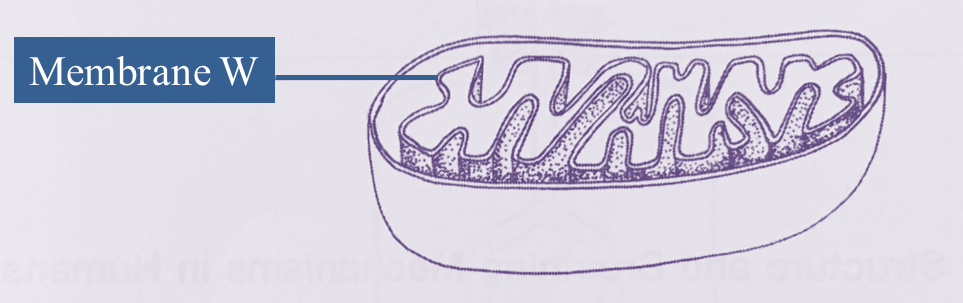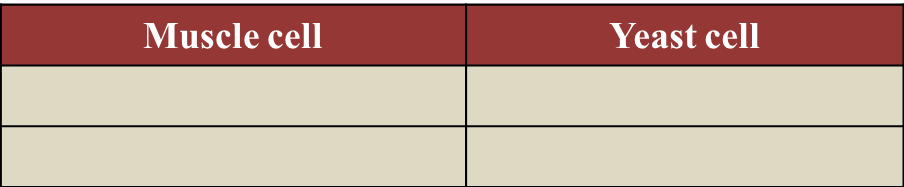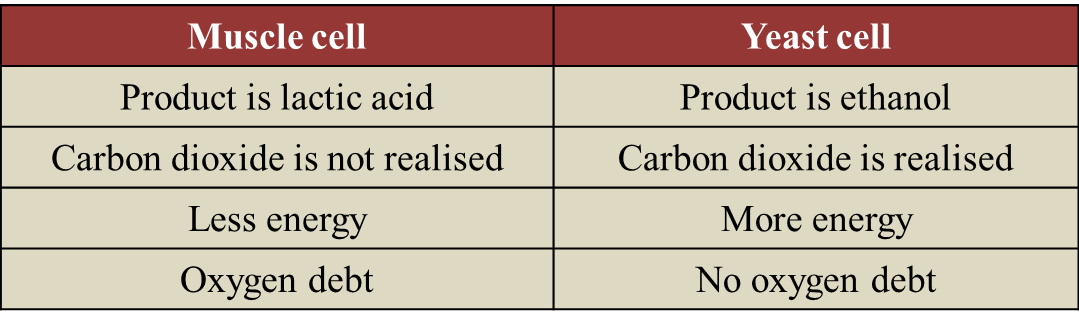# 7.7.1 The Respiratory Process in Energy Production (Structured Question 1 & 2)

Question 1:
Diagram below shows a type of organelle found in muscle cells.
A biochemical reaction occurs in the organelle.(a)(i)
Name this organelle.
(ii) Explain why membrane W is in the form of numerous folded layers.
(b) The biochemical reaction that occurs in this organelle is summarized as follows:(i) Name gas R.
(ii) Name product S.
(iii) Explain why the muscle cell has a large number of these organelles.
(iv) If the blood sugar level is lower than the normal range, the biochemical reaction in this organelle can still occur.
Explain how.
(c) State two differences on the biochemical reaction occurring between muscle cell and yeast cell in the absence of gas R.(a)(i)
Mitochondrion

(a)(ii)
Membrane W is in the form of numerous folded layers to increase total surface area, for the efficiency of cellular respiration.

(b)(i)
Gas R is oxygen

(b)(ii)
Product S is water

(b)(iii)
Muscle cells need a large number of mitochondrion as an energy ATP supply in order to oxidise glucose in aerobic respiration.

(b)(iv)
– If the blood sugar is lower than the normal range, less energy is produced.
– Pancreas secretes glucagon to convert glycogen to glucose.
– Cellular respiration occurs.

(c)